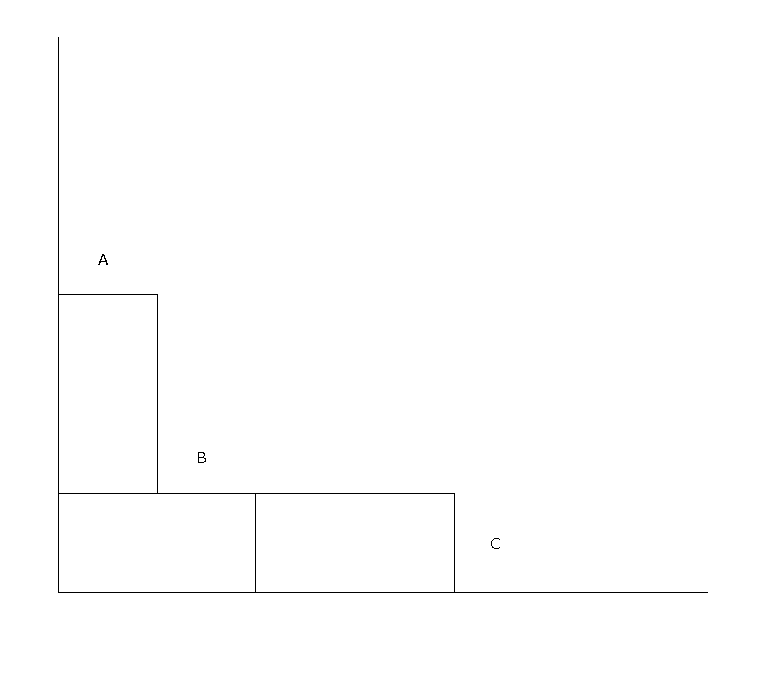Stochastic Integrable Systems

Supervisors: Nikolaos Zygouras, Yacine Barhoumi

Our research study group has been focused on studying domino growth systems and their relations to random matrices. Here is a summary on some basic objects involved. We defined the growth model as follows:

Given a parameter$q \in [0,1]$ and a certain configuration of dominoes we look at where new dominoes can be added. See the picture for an example of admissable positions, which are the places where a domino can be inserted without overhanging. These are denoted by A, B and C. (More precisely they are the positions where adding a domino will still leave a domino diagram.)Let S be the set of locations where a domino can be added (if two dominoes can be added in one site, we include this site twice in S. In the figure above, position B will need to be counted twice as both a horizontal and a vertical domino may be added.). Assign to each element of S an exponential clock. When the clock rings, the probability of the corresponding domino being included depends on its orientation. If the domino that can be added at the site has horizontal orientation, then add it to the cluster. If the domino has vertical orientation, then add it with probability$q$. Then repeat the process.

We created some matlab code that ran simulations for this model. You can see the results here. Below is a video showing how the model develops with$q=0.5$

The main reason we study this model is because it is a modification of the corner growth model, outlined in [Joh00],which belongs to the KPZ universality class. To be more precise, suppose that we rotate the picture above by 45 degrees anticlockwise (so that the two axis are now represented by the graph of the function h(x)=|x|. Now, suppose that we want to study the behaviour of the line that separates the area that is covered by tiles and the area above that is not covered yet (as in the next picture). Now, we let the size of the tiles to go to 0 and then the line we are interested in becomes a macroscopic rough object. It turns out that, if h(t,x) is the height of this line at time t and above the position that is at horizontal distance x from the origin, then h solves the KPZ equation$$\partial_th=v\partial_x^2h+\lambda(\partial_xh)^2+\eta$$

As the corner growth model has many results already proven, we suspected that we could adapt some of the proofs to the domino case. Integral to the CGM were the schur polynomials. These results were found by representing the CGM as a random matrix with entries that follow Geometric Distributions. Then, one can use an algorithm called RSK correspondence and connect these kinds of matrices with Schur polynomials, which makes the model easier to handle since it can be represented as a Determinantal Point Process ( [Joh00] contains more details).

The analogue of the Schur polynomials in the domino case are the LLT polynomials. You can find more detailed information on them here. They are symmetric polynomials that are related to domino tableaux and are related to the spin of a particular tiling (half the number of vertical dominoes. A crucial fact about them is that they satisfy the Cauchy identity. If D is the set of all domino tableaux, then the Cauchy identity states:$$\sum_{\lambda \in \mathcal{D}} G_{\lambda}(X, q^{\frac{1}{2}}) G_{\lambda}(Y, q^{\frac{1}{2}}) = \prod_{i,j} \prod_{k=0}^1 \frac{1}{1-q^kX_iY_j}$$

This allows us to define a probability measure on the set of all domino tableaux, using the LLT polynomials, using the Cauchy identity to normalise appropriately.$$\mathbb{P}_{\rho_1,\rho_2;q}(\lambda)=\dfrac{G_\lambda(\rho_1,q^{\frac{1}{2}})G_\lambda(\rho_2,q^{\frac{1}{2}})}{\bar{H}(\rho_1;\rho_2)}$$

Our main goal was to adapt the proofs of the CGM for the Domino Case. Although, we can connect the LLT polynomials to a pair of matrices with entries that follow a Geometric distribution, we were not able to fully represent the Domino model in terms of pairs of matrices like that (unlike CGM which is represented as a single matrix of this form). Furthermore, if there exists a connection between LLT polynomials and Determinantal Point Processes (as in the CGM where we had Schur polynomials) it is not that easy to find and prove it.

References

[Joh00] Kurt Johansson. “Shape fluctuations and random matrices”. In: Comm. Math. Phys. 209.2 (2000)

Acknowledgements

We would like to thank our supervisors Nikos Zygouras and Yacine Barhoumi for suggesting the problem and for helpful discussions. We also acknowledge the funding body EPSRC and the support from MASDOC CDT.